# Solution assignment 04 Quadratic functions and graphs

### Assignment 4

The equation of a parabola is: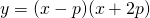For which value of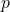does the top of this parabola lie on the line:### Solution

The coordinates of the intersection points of this parabola with the-axis are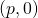and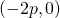. The symmetry axis lies just in the middle between these two points and thus we have: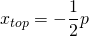The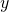-coordinate of the top is: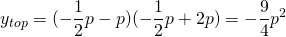The top lies on the lineso we have: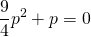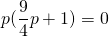and thus: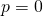or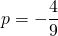0